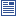Accessibility options:

# Differential Equations resources

Show me all resources applicable to

### Community Project (3)Mathematical Tools for Physical Sciences and Systems Biology (SOURCE)
A zip file containing the LaTex source files and metatdata for the leaflet - a summary of common mathematical definitions and properties used in the Physical Sciences and Systems Biology contributed to the mathcentre Community Project by Dr Morgiane Richard, University of Aberdeen and reviewed by Mamen Romano and Ian Stansfield, University of Aberdeen.Solving Differential Equations by Separating Variables SOURCE
A zip file containing LaTeX source and eps files for the quick reference leaflet 'Solving Differential Equations by Separating Variables' contributed to the mathcentre Community Project by Katy Dobson and reviewed by Alan Slomson, University of Leeds.Solving Differential Equations with Integrating Factors SOURCE
A zip file containing LaTeX source and eps files for the quick reference leaflet 'Solving Differential Equations with Integrating Factors' contributed to the mathcentre Community Project by Katy Dobson and reviewed by Alan Slomson, University of Leeds.

### Facts & Formulae Leaflets (1)Mathematical Tools for Physical Sciences and Systems Biology
This leaflet is a summary of common mathematical definitions and properties used in the Physical Sciences and Systems Biology contributed to the mathcentre Community Project by Dr Morgiane Richard, University of Aberdeen and reviewed by Mamen Romano and Ian Stansfield, University of Aberdeen.

### Quick Reference (2)Solving Differential Equations by Separating Variables
Quick Reference leaflet on first order differential equations. This Quick Reference leaflet is contributed to the mathcentre Community Project by Katy Dobson and reviewed by Alan Slomson, University of Leeds.Solving Differential Equations with Integrating Factors
Quick Reference leaflet on solving differential equations. This Quick Reference leaflet is contributed to the mathcentre Community Project by Katy Dobson and reviewed by Alan Slomson, University of Leeds.

### Teach Yourself (1)First Order Ordinary Differential Equations
First Order Differential Equations: A summary of five common methods to solve first order ODEs: direct integration, separation of variables, homogeneous equations, integrating factor and Bernouilli equations. This Teach Yourself resource is contributed to the mathcentre Community Project by Morgiane Richard, University of Aberdeen and is reviewed by Shazia Ahmed, University of Glasgow.

### Test Yourself (2)Differential Equations Test 01 (DEWIS)
Four questions on second order linear constant coefficient differential equations. The first two involve identifying the complementary function, the third involves applying initial conditions and the fourth involves finding a particular solution with either linear or sinusoidal forcing. DEWIS resources have been made available under a Creative Commons licence by Rhys Gwynllyw & Karen Henderson, University of the West of England, Bristol.First order differential equations - Numbas
9 questions on first order differential equations. Straight forward integration (2), separating variables (4), linear (1), homogenous (2). All are either initial value or boundary value problems. Numbas resources have been made available under a Creative Commons licence by Bill Foster and Christian Perfect, School of Mathematics & Statistics at Newcastle University.